Next: WKB Approximation Up: Wave Propagation in Inhomogeneous Previous: Reflection by Conducting Surfaces

Let us investigate the propagation of an electromagnetic wave though a spatially non-uniform dielectric medium. As a specific example, consider the propagation of radio waves through the Earth's ionosphere. The refractive index of the ionosphere can be written [see Equation (801)]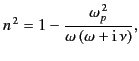(1054)

where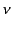is a real positive constant that parameterizes the damping of electron motion (in fact,is the collision frequency of free electrons with other particles in the ionosphere), and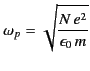(1055)

is the plasma frequency. In the previous formula,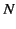is the density of free electrons in the ionosphere, and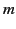is the electron mass. We shall assume that the ionosphere is horizontally stratified, so that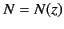, where the coordinate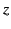measures height above the Earth's surface (the curvature of the Earth's surface is neglected in the following analysis). The ionosphere actually consists of two main layers; the E-layer, and the F-layer. We shall concentrate on the lower E-layer, which lies about 100 km above the surface of the Earth, and is about 50 km thick. The typical day-time number density of free electrons in the E-layer is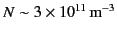. At night-time, the density of free electrons falls to about half this number. The typical day-time plasma frequency of the E-layer is, therefore, about 5 MHz. The typical collision frequency of free electrons in the E-layer is about 0.05 MHz. According to simplistic theory, any radio wave whose frequency lies below the day-time plasma frequency, 5 MHz, (i.e., any wave whose wavelength exceeds about 60 m) is reflected by the ionosphere during the day. Let us investigate in more detail how this process takes place. Note, incidentally, that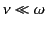for mega-Hertz frequency radio waves, so it follows from Equation (1056) that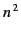is predominately real (i.e., under normal circumstances, electron collisions can be neglected).

The problem of radio wave propagation through the ionosphere was of great practical importance during the first half of the 20th century, because, during that period, long-wave radio waves were the principal means of military communication. Nowadays, the military have far more reliable methods of communication. Nevertheless, this subject area is still worth studying, because the principal tool used to deal with the problem of wave propagation through a non-uniform medium--the so-called WKB approximation--is of great theoretical importance. In particular, the WKB approximation is very widely used in quantum mechanics (in fact, there is a great similarity between the problem of wave propagation through a non-uniform medium, and the problem of solving Schrödinger's equation in the presence of a non-uniform potential).

Maxwell's equations for a wave propagating through a non-uniform, unmagnetized, dielectric medium are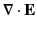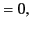(1056)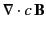(1057)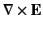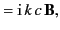(1058)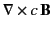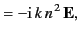(1059)

where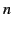is the non-uniform refractive index of the medium. It is assumed that all field quantities vary in time like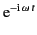, where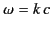. Note that, in the following,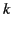is the wavenumber in free space, rather than the wavenumber in the dielectric medium.Next: WKB Approximation Up: Wave Propagation in Inhomogeneous Previous: Reflection by Conducting Surfaces
Richard Fitzpatrick 2014-06-27